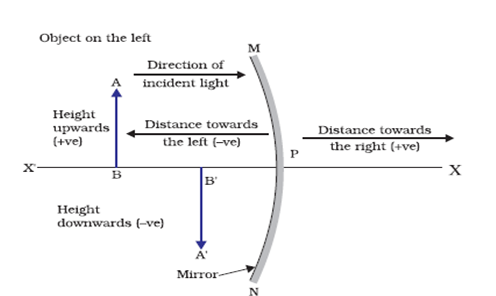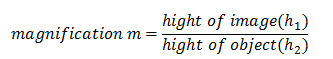# Reflection of light Notes for Class 10

## Sign convention for reflection by spherical mirrors

Reflection of light by spherical mirrors follow a set of sign conventions called the New Cartesian Sign Convention. In this convention, the pole (P) of the mirror is taken as the origin. The principal axis of the mirror is taken as the x-axis (X�X) of the coordinate system. The conventions are as follows �
• The object is always placed to the left of the mirror. This implies that the light from the object falls on the mirror from the left-hand side.
• All distances parallel to the principal axis are measured from the pole of the mirror.
• All the distances measured to the right of the origin (along + x-axis) are taken as positive while those measured to the left of the origin (along � x-axis) are taken as negative.
• Distances measured perpendicular to and above the principal axis (along + y-axis) are taken as positive.
• Distances measured perpendicular to and below the principal axis (along �y-axis) are taken as negative.
These new Cartesian sign convention for spherical mirrors are shown below in the figure## Mirror formula and magnification

### Mirror formula:-

It gives the relationship between image distance (v) , object distance (u) and the focal length (f) of the mirror and is written as
$\frac{1}{v} + \frac{1}{u} = \frac{1}{f}$
Where v is the distance of image from the mirror, u is the distance of object from the mirror and f is the focal length of the mirror. This formula is valid in all situations for all spherical mirrors for all positions of the object.

### Magnification

agnification produced by a spherical mirror gives the relative extent to which the image of an object is magnified with respect to the object size. It is expressed as the ratio of the height of the image to the height of the object. It is usually represented by the letter m. So,or,
$m = \frac{{{h_1}}}{{{h_2}}}$
The magnification m is also related to the object distance (u) and image distance (v) and is given as
$m = \frac{{{h_1}}}{{{h_2}}} = - \frac{v}{u}$

Given below are the links of some of the reference books for class 10 Science.

You can use above books for extra knowledge and practicing different questions.

### Practice Question

Question 1 Which among the following is not a base?
A) NaOH
B) $NH_4OH$
C) $C_2H_5OH$
D) KOH
Question 2 What is the minimum resistance which can be made using five resistors each of 1/2 Ohm?
A) 1/10 Ohm
B) 1/25 ohm
C) 10 ohm
D) 2 ohm
Question 3 Which of the following statement is incorrect? ?
A) For every hormone there is a gene
B) For production of every enzyme there is a gene
C) For every molecule of fat there is a gene
D) For every protein there is a gene

Note to our visitors :-

Thanks for visiting our website.